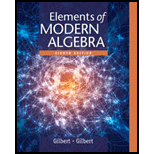# Suppose the alphabet consists of a through z , in natural order, followed by a blank and then the digits 0 through 9 , in natural order. Associate these "letters" with the numbers 0 , 1 , 2 , ... , 36 , respectively, thus forming a 37 -letter alphabet, D . Use the affine cipher to decipher the message X 0 1 9 1 6 R 9 1 6 5 4 6 M 9 C N 1L 6 B1 L L 6 X 0 R Z 6 U I I if you know that the plaintext message begins with " t " followed by " h ". Write out the affine mapping f and its inverse.### Elements Of Modern Algebra

8th Edition
Gilbert + 2 others
Publisher: Cengage Learning,
ISBN: 9781285463230### Elements Of Modern Algebra

8th Edition
Gilbert + 2 others
Publisher: Cengage Learning,
ISBN: 9781285463230

#### Solutions

Chapter
Section
Chapter 2.8, Problem 11E
Textbook Problem

## Suppose the alphabet consists of a through z , in natural order, followed by a blank and then the digits 0 through   9 , in natural order. Associate these "letters" with the numbers 0 ,   1 ,   2 ,   ...   ,   36 , respectively, thus forming a 37 -letter alphabet, D . Use the affine cipher to decipher the message X 0 1 9 1 6 R 9 1 6 5 4 6 M 9   C N   1L 6 B1   L   L   6   X   0   R Z   6 U   I   I   if you know that the plaintext message begins with " t " followed by " h ". Write out the affine mapping f and its inverse.

Expert Solution
To determine

Plain text in the 37 letter alphabet D, use the affine cipher to decipher the message “ X01916R916546M9CN1L6B1LL6X0RZ6UII” and if the plain text message begins with “t” followed by “h”. Write down the affine mapping f and f1.

### Explanation of Solution

Given information:

The 37-letter alphabet D -

If alphabet consist of a to z, in natural order followed by blank and then digits through 0to9, then have 37 “letters” that associates with the integer 0,1,2,3,...,35 as follows:

Alphabet: abcdef...vwxyz"blank"012...9

D:012345...212223242526272829...35

Formula used:

(i) Affine cipher:

Define the mapping f:AA by f(x)=ax+bmodn, where a,b are fixed integers and order pair a,b forms a key to affine cipher.

(ii) Euclidean Algorithm:

Let a and b are integers with b>0, then the procedure

a=bq0+r10r1<bb=r1q1+r20r2<r1r1=r2q2+r30r3<r2rk=rk+1qk+1+rk+20rk+2<rk+1

is known as Euclidean Algorithm.

Since the integers r1,r2,,rk+2 are decreasing and are all non-negative, there is a smallest integer n such that rn+1=0:

rn1=rnqn+rn+10=rn+1

If r0=b then the last non-zero remainder rn is always greatest common divisor of a and b.

Calculation:

It seems reasonable that the cipher-text letters x and o corresponds to the plaintext t and h.

Translating these into the set D,

xtranslatetoD23ttranslatetoD19

otranslatetoD27htranslatetoD7

Now, to determine the key from the following system of equations for aandb

23=a(19)+bmod3727=a(7)+bmod37

Use a=b(modn)b=a(modn)

a(19)+b=23mod37…… (1)

a(7)+b=27mod37 …… (2)

Subtract (1) from (2), gives

a(7)a(19)=2723mod37

a(12)=4mod37

a(25)=4mod37 as 25=12(mod37)

25a=4mod37

Now to find the multiplicative inverse using Euclidean algorithm.

25=12(2)+1

Thus,

1=2512(2)

1=25(2)(3725)

1=2537(2)+25(2)

1=25(3)37(2)

Thus, 25(3)=1(mod37)

The inverse 251=3(mod37).

Hence, 25a=4mod37

a=2514(mod37)

a=(3)4(mod37)

a=12(mod37)

Now, from (1), a(19)+b=23mod37

(12(mod37))(19)+b=23mod37

b=12(mod37)19+23(mod37)

b=12(19)+23(mod26)

b=228+23(mod37)

b=205(mod37)

b=17(mod37) as 17=205(mod37)

Therefore, a=12 and b=17

Now, to define affine mapping as, f:DD such that, f(x)=12x+17mod37, where D={0,1,2,3,

### Want to see the full answer?

Check out a sample textbook solution.See solution

### Want to see this answer and more?

Bartleby provides explanations to thousands of textbook problems written by our experts, many with advanced degrees!

See solution